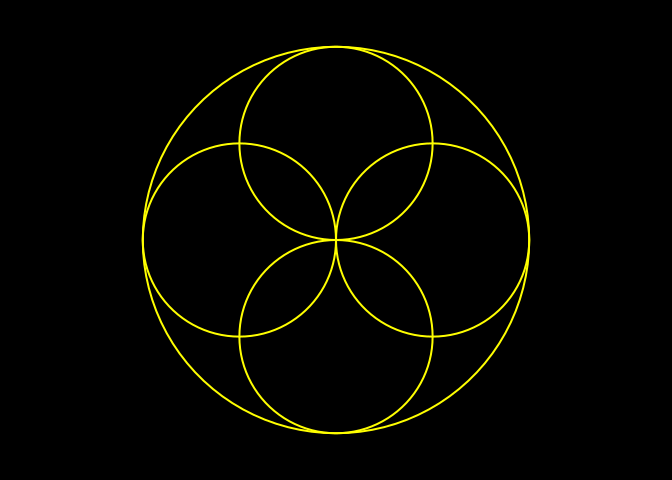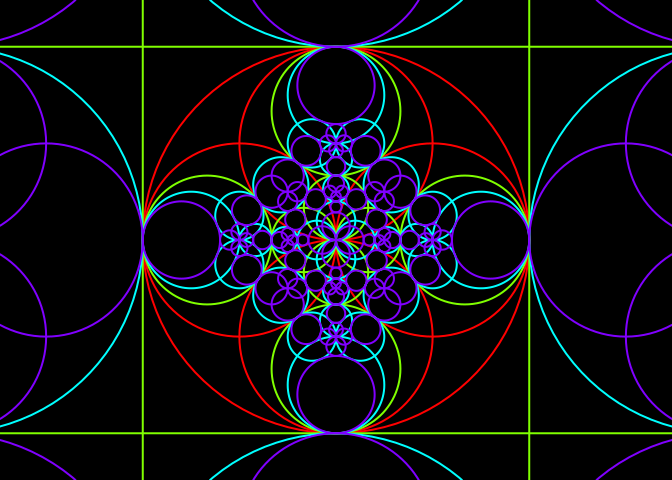# Generalized circles and inversions

Posted on March 13, 2019 by Stéphane Laurent
Tags: R, maths, geometry

Let $$\iota_0$$ be the inversion with respect to the unit circle: $\iota_0(z) = \frac{1}{\bar{z}}, \quad z \neq 0.$

Consider a generalized circle $$\mathcal{G}\mathcal{C}$$ having equation $\boxed{Az\bar{z} + \bar{\gamma}z + \gamma\bar{z} = D}$ which is equivalent, by dividing by $$z\bar{z}$$, to $A + \bar{\gamma}\frac{1}{\bar{z}} + \gamma\frac{1}{z} = \frac{D}{z\bar{z}}.$

Plugging $$z = \iota_0^{-1}(w) = 1/\bar{w}$$ into this equation, we get the following equation of $$\iota_0(\mathcal{G}\mathcal{C})$$: $A + \bar{\gamma}w + \gamma\bar{w} = D w\bar{w} \\ \iff \, \boxed{D w\bar{w} - \bar{\gamma}w - \gamma\bar{w} = A}.$ This is the equation of a generalized circle.

Now consider the inversion $$\iota$$ with respect to the circle of center $$c$$ and radius $$r$$: $\iota(z) = c + \frac{r^2}{\overline{z-c}}, \quad z \neq c.$

One has $\iota = f^{-1} \circ \iota_0 \circ f$ where $$f(z) = \frac{z-c}{r}$$, and $$f^{-1}(z) = rz + c$$.

The transformation $$f$$ is a “scaling-translation”. It is clear that it maps a generalized circle to a generalized circle, and this also holds for $$f^{-1}$$. Therefore, $$\iota$$ maps any generalized circle to a generalized circle. This result is well-known. Here, we will give the equation of the image generalized circle.

# Case when $$\mathcal{G}\mathcal{C}$$ is a circle

Assume that $$\mathcal{G}\mathcal{C}$$ is the circle $$\mathcal{C}_0$$ with center $$z_0$$ and radius $$R_0$$. Then $$f(\mathcal{C_0})$$ is the circle $$\mathcal{C_1}$$ with center $$z_1 = \frac{z_0-c}{r}$$ and radius $$R_1 = \frac{R_0}{r}$$. It has equation $z\bar{z} - \bar{z_1}z - z_1\bar{z} = D_1$ with $$D_1 = R_1^2-{|z_1|}^2$$.

Then $$\iota_0(\mathcal{C_1})$$ is the generalized circle having equation $D_1 z\bar{z} + \bar{z_1}z + z_1\bar{z} = 1.$

### Case $$D_1 \neq 0$$

If $$D_1 \neq 0$$, the above equation of $$\iota_0(\mathcal{C_1})$$ is the equation of a circle $$\mathcal{C}_2$$ with center $$z_2 = -\frac{z_1}{D_1}$$ and radius $R_2 = \sqrt{\frac{{|z_1|}^2}{D_1^2} + \frac{1}{D_1}}.$

Now, $$f^{-1}(\mathcal{C}_2) = \iota(\mathcal{C}_0)$$ is the circle with center $$r z_2 + c$$ and radius $$r R_2$$.

### Case $$D_1 = 0$$

If $$D_1=0$$, our equation of $$\iota_0(\mathcal{C_1})$$ is $\bar{z_1}z + z_1\bar{z} = 1,$ which is the equation of a line $$\mathcal{L_2}$$, with direction $$\theta_2 = \textrm{arg}(z_1)$$ and offset $$d_2 = \frac{1}{2{|z_1|}^2}$$.

Then $$f^{-1}(\mathcal{L}_2) = \iota(\mathcal{C}_0)$$ is a line parallel to $$\mathcal{L}_2$$, that is, its direction is $$\theta_2$$. To get its offset, take a point $$w_2$$ on $$\mathcal{L}_2$$: $w_2 = \begin{cases} \dfrac{1}{2 \Re(z_1)} & \text{if } \Im(z_1) = 0 \\ \dfrac{i}{2 \Im(z_1)} & \text{if } \Im(z_1) \neq 0 \end{cases}$ and take its image $$w_3 = f^{-1}(w_2) = r w_2 + c$$. Then the offset is $\cos(\theta_2) \Re(w_3) + \sin(\theta_2) \Im(w_3) \\ \qquad = \frac{\Re(z_1)}{|z_1|}\Re(w_3) + \frac{\Im(z_1)}{|z_1|}\Im(w_3).$

# Case when $$\mathcal{G}\mathcal{C}$$ is a line

Now assume that $$\mathcal{G}\mathcal{C}$$ is the line $$\mathcal{L}_0$$ with direction $$\theta_0$$ and offset $$d_0$$. Its image $$f(\mathcal{L}_0)$$ is a line $$\mathcal{L}_1$$ parallel to $$\mathcal{L}_0$$, that is the direction of $$\mathcal{L}_1$$ is $$\theta_0$$. To get its offset, take a point $$w_0$$ on $$\mathcal{L}_0$$: $w_0 = \begin{cases} \dfrac{d_0}{\cos(\theta_0)} & \text{if } \sin(\theta_0) = 0 \\ i\dfrac{d_0}{\sin(\theta_0)} & \text{if } \sin(\theta_0) \neq 0 \end{cases}$ and take its image $$w_1 = f(w_0) = \frac{w_0-c}{r}$$. Then the offset of $$\mathcal{L}_1$$ is $d_1 = \cos(\theta_0) \Re(w_1) + \sin(\theta_0) \Im(w_1)$ and the line $$\mathcal{L}_1$$ has equation $\bar{\gamma_1}z + \gamma_1\bar{z} = D_1$ with $$\gamma_1 = \cos(\theta_0) + i\sin(\theta_0)$$ and $$D_1 = 2d_1$$.

Therefore, $$\iota_0(\mathcal{L}_1)$$ is the generalized circle having equation $D_1 z\bar{z} - \bar{\gamma_1}z - \gamma_1\bar{z} = 0.$

### Case $$D_1 \neq 0$$

If $$D_1 \neq 0$$, then $$\iota_0(\mathcal{L}_1)$$ is the circle $$\mathcal{C}_2$$ with center $$z_2 = \frac{\gamma_1}{D_1}$$ and radius $$R_2 = \frac{|\gamma_1|}{|D_1|}$$. Then $$f^{-1}(\mathcal{C}_2) = \iota(\mathcal{L}_0)$$ is the circle with center $$r z_2 + c$$ and radius $$r R_2$$.

### Case $$D_1 = 0$$

If $$D_1 = 0$$, then $$\iota_0(\mathcal{L}_1)$$ is the line $$\mathcal{L}_2$$ having equation $\bar{\gamma_1}z + \gamma_1\bar{z} = 0.$ Its direction is $$\textrm{arg}(\gamma_1) = \theta_0$$ and its offset is $$0$$.

Then $$f^{-1}(\mathcal{L}_2) = \iota(\mathcal{C}_0)$$ is a line $$\mathcal{L}_3$$ parallel to $$\mathcal{L}_2$$, that is, its direction is $$\theta_0$$. The line $$\mathcal{L}_2$$ passes through $$0$$, therefore the point $$f^{-1}(0) = c$$ belongs to $$\mathcal{L}_3$$. Consequently, the offset of $$\mathcal{L}_3$$ is $\cos(\theta_0) \Re(c) + \sin(\theta_0) \Im(c).$

# R code

We firstly write a function iotaCircle which calculates the image of a circle.

# squared modulus
Mod2 <- function(z){
Re(z)^2 + Im(z)^2
}
# image of a circle
iotaCircle <- function(c, r, circle, tol = sqrt(.Machine$double.eps)){ z0 <- circle$center; R0 <- circle$radius z1 <- (z0-c)/r D1 <- (R0/r)^2 - Mod2(z1) if(abs(D1) > tol){ z2 <- -z1/D1 R2 <- sqrt(Mod2(z2) + 1/D1) out <- list(center = r*z2+c, radius = r*R2) attr(out, "type") <- "circle" }else{ theta2 <- Arg(z1) %% (2*pi) w2 <- ifelse(Im(z1) == 0, 1/2/Re(z1), 1i/2/Im(z1)) w3 <- r*w2 + c out <- list( theta = theta2, offset = (Re(z1)*Re(w3)+Im(z1)*Im(w3))/Mod(z1) ) attr(out, "type") <- "line" } out } Let’s check the function iotaCircle. To do so, we use the circumcircle function below, which returns the center and the radius of the circle passing by z1, z2, z3. circumcircle <- function(z1,z2,z3){ x1 <- Re(z1); y1 <- Im(z1); p1 <- c(x1,y1) x2 <- Re(z2); y2 <- Im(z2); p2 <- c(x2,y2) x3 <- Re(z3); y3 <- Im(z3); p3 <- c(x3,y3) a <- det(cbind(rbind(p1,p2,p3),1)) q1 <- c(crossprod(p1)); q2 <- c(crossprod(p2)); q3 <- c(crossprod(p3)) q <- c(q1,q2,q3) x <- c(x1,x2,x3) y <- c(y1,y2,y3) Dx <- det(cbind(q,y,1)) Dy <- -det(cbind(q,x,1)) c <- det(cbind(q,x,y)) center <- c(Dx,Dy)/a/2 r <- sqrt(c(crossprod(center-p1))) list(center = center + 1i*center, radius = r) } Now let’s check iotaCircle. # define iota c <- 1 + 2i; r <- 3 iota <- function(z){ c + r^2/Conj(z-c) } # define C0 - a case when iota(C0) is a circle #### z0 <- 2 + 3i; R0 <- 2 iotaCircle(c, r, circle = list(center = z0, radius = R0)) ##$center
##  -3.5-2.5i
##
## $radius ##  9 ## ## attr(,"type") ##  "circle" circumcircle(iota(z0+R0), iota(z0-R0), iota(z0+1i*R0)) ##$center
##  -3.5-2.5i
##
## radius ##  9 # now, a case when iota(C0) is a line #### R0 <- sqrt(2) w1 <- iota(z0+R0); w2 <- iota(z0-R0); w3 <- iota(z0+1i*R0) (w2-w1)/(w3-w1) # aligned <=> Im = 0 ##  3.414214+0i (L <- iotaCircle(c, r, circle = list(center = z0, radius = R0))) ##theta
##  0.7853982
##
## $offset ##  5.303301 ## ## attr(,"type") ##  "line" cos(L$theta)*Re(w1) + sin(L$theta)*Im(w1) # = L$offset
##  5.303301
cos(L$theta)*Re(w2) + sin(L$theta)*Im(w2) # = L$offset ##  5.303301 cos(L$theta)*Re(w3) + sin(L$theta)*Im(w3) # = L$offset
##  5.303301

Now we write a function iotaLine which calculates the image of a line.

iotaLine <- function(c, r, line, tol = sqrt(.Machine$double.eps)){ theta0 <- line$theta; d0 <- line$offset w0 <- ifelse(sin(theta0) == 0, d0/cos(theta0), 1i*d0/sin(theta0)) w1 <- (w0-c)/r D1 <- 2 * (cos(theta0)*Re(w1) + sin(theta0)*Im(w1)) if(abs(D1)>tol){ gamma1 <- cos(theta0) + 1i*sin(theta0) z2 <- gamma1/D1 R2 <- Mod(gamma1/D1) out <- list(center = r*z2+c, radius = r*R2) attr(out, "type") <- "circle" }else{ out <- list( theta = theta0, offset = cos(theta0)*Re(c)+sin(theta0)*Im(c) ) attr(out, "type") <- "line" } out } Let’s check iotaLine. Firstly, a case when the image is a circle. # define L0 theta0 <- 1; d0 <- 2 theta0 <- pi/4; d0 <- 0 # take three points on iota(L0) x <- c(0,1,2) y <- (d0 - cos(theta0)*x) / sin(theta0) w1 <- iota(x + 1i*y) w2 <- iota(x + 1i*y) w3 <- iota(x + 1i*y) # check circumcircle(w1,w2,w3) ##$center
##  -0.5+0.5i
##
## $radius ##  2.12132 iotaLine(c, r, list(theta = theta0, offset = d0)) ##$center
##  -0.5+0.5i
##
## radius ##  2.12132 ## ## attr(,"type") ##  "circle" Now a case when the image is a line. # define iota c <- 1-1i iota <- function(z){ c + r^2/Conj(z-c) } # define L0 theta0 <- pi/4; d0 <- 0 # take three points on iota(L0) x <- c(0,2,4) y <- (d0 - cos(theta0)*x) / sin(theta0) w1 <- iota(x + 1i*y) w2 <- iota(x + 1i*y) w3 <- iota(x + 1i*y) # check (w2-w1)/(w3-w1) # aligned <=> Im = 0 ##  1.5+0i ( L <- iotaLine(c, r, list(theta = theta0, offset = d0)) ) ##theta
##  0.7853982
##
## $offset ##  1.110223e-16 ## ## attr(,"type") ##  "line" cos(L$theta)*Re(w1) + sin(L$theta)*Im(w1) # = L$offset
##  -4.440892e-16
cos(L$theta)*Re(w2) + sin(L$theta)*Im(w2) # = L$offset ##  -4.440892e-16 cos(L$theta)*Re(w3) + sin(L$theta)*Im(w3) # = L$offset
##  -4.440892e-16

Finally, let’s put things together in a single function.

iotaGcircle <- function(c, r, gcircle){
if(attr(gcircle, "type") == "circle"){
iotaCircle(c, r, gcircle)
}else{
iotaLine(c, r, gcircle)
}
}

# Illustration

We will make a beautiful picture of inverted circles. This is borrowed from this circle inversion gallery.

Firstly, let’s write a function which draws a generalized circle. We use the draw.circle function of the plotrix package.

library(plotrix)
drawLine <- function(line, ...){
theta <- line$theta; offset <- line$offset
if(sin(theta) != 0){
abline(a = offset/sin(theta), b = -1/tan(theta), ...)
}else{
abline(v = offset/cos(theta), ...)
}
}
drawCircle <- function(circle, ...){
draw.circle(Re(circle$center), Im(circle$center), circle$radius, ...) } drawGcircle <- function(gcircle, color = "black", ...){ if(attr(gcircle, "type") == "circle"){ drawCircle(gcircle, border = color, ...) }else{ drawLine(gcircle, col = color, ...) } } We start with five circles. # generation 0 gen0 <- sapply(c(0, pi/2, pi, 3*pi/2), function(beta){ out <- list(center = cos(beta)+1i*sin(beta), radius = 1, gen = 0) attr(out, "type") <- "circle" out }, simplify = FALSE) gen0[] <- list(center = 0+0i, radius = 2, gen = 0) attr(gen0[], "type") <- "circle" # plot par(bg = "black", mar = c(0,0,0,0)) plot(0, 0, type="n", xlim=c(-2.3,2.3), ylim=c(-2.3,2.3), asp=1, axes=FALSE, xlab=NA, ylab=NA) invisible(lapply(gen0, drawGcircle, color = "yellow", lwd = 2))Now, we invert each of these five circles with respect to the other ones: # generation 1 n0 <- length(gen0) n1 <- n0*(n0-1) gen1 <- vector("list", n1) k <- 0 while(k < n1){ for(j in 1:n0){ for(i in 1:n0){ if(i != j){ k <- k+1 gen1[[k]] <- iotaGcircle(gen0[[i]]$center, gen0[[i]]$radius, gen0[[j]]) gen1[[k]]$base <- i
gen1[[k]]$gen <- 1 } } } } We continue so on: we invert the obtained generalized circles with respect to the starting circles. # generation 2 n2 <- n0*n1-n1 gen2 <- vector("list", n2) k <- 0 while(k < n2){ for(j in 1:n1){ for(i in 1:n0){ if(gen1[[j]]$base != i){
k <- k+1
gen2[[k]] <-
iotaGcircle(gen0[[i]]$center, gen0[[i]]$radius, gen1[[j]])
gen2[[k]]$base <- i gen2[[k]]$gen <- 2
}
}
}
}
# generation 3
n3 <- n0*n2-n2
gen3 <- vector("list", n3)
k <- 0
while(k < n3){
for(j in 1:n2){
for(i in 1:n0){
if(gen2[[j]]$base != i){ k <- k+1 gen3[[k]] <- iotaGcircle(gen0[[i]]$center, gen0[[i]]$radius, gen2[[j]]) gen3[[k]]$gen <- 3
}
}
}
}

Let’s put all the obtained generalized circles together:

gcircles <- c(gen0, gen1, gen2, gen3)
length(gcircles)
##  425

There are 425 generalized circles, but some of them are duplicated. To remove the duplicates, we use the uniqueWith function below. This function takes as arguments an atomic vector or a list v and a function f of two arguments, two elements of v, and which returns TRUE or FALSE, according to whether the two elements are considered as duplicates.

uniqueWith <- function(v, f){
size <- length(v)
for(i in seq_len(size-1L)){
j <- i + 1L
while(j <= size){
if(f(v[[j]],v[[i]])){
v <- v[-j]
size <- size - 1L
}else{
j <- j + 1L
}
}
}
v[1L:size]
}
# examples ####
v <- c(1,1,2,1,3,4,3,5,5)
uniqueWith(v, ==)
##  1 2 3 4 5
uniqueWith(v, function(x,y) (x-y) %% 3 == 0)
##  1 2 3
v <- list(a="you", b="are", c="great")
uniqueWith(v, function(x,y) nchar(x) == nchar(y))
## $a ##  "you" ## ##$c
##  "great"

So let’s define the function f which identifies two equal generalized circles.

f <- function(gcircle1, gcircle2){
if(attr(gcircle1, "type") == attr(gcircle2, "type")){
if(attr(gcircle1, "type") == "circle"){
Mod(gcircle1$center-gcircle2$center) < 1e-5 &&
abs(gcircle1$radius-gcircle2$radius) < 1e-5
}else{
abs(cos(gcircle1$theta)-cos(gcircle2$theta)) < 1e-5 &&
abs(sin(gcircle1$theta)-sin(gcircle2$theta)) < 1e-5 &&
abs(gcircle1$offset-gcircle2$offset) < 1e-5
}
}else{
FALSE
}
}

And now, let’s remove the duplicates:

gcircles <- uniqueWith(gcircles, f)
length(gcircles)
##  161

Now we plot the generalized circles, with a color indicating the generation.

draw <- function(gcircle, colors=rainbow(4), ...){
drawGcircle(gcircle, color = colors[1+gcircle\$gen], ...)
}
par(mar = c(0,0,0,0), bg = "black")
plot(0, 0, type="n", xlim=c(-2.3,2.3), ylim=c(-2.3,2.3),
asp=1, axes=FALSE, xlab=NA, ylab=NA)
invisible(lapply(gcircles, draw, lwd=2))